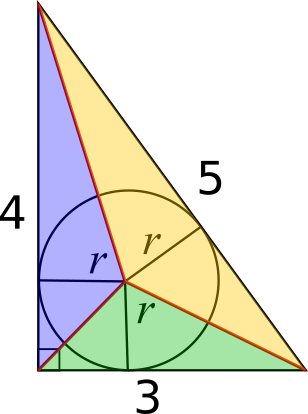#### You may also like### Some(?) of the Parts

A circle touches the lines OA, OB and AB where OA and OB are perpendicular. Show that the diameter of the circle is equal to the perimeter of the triangle### Triangle Midpoints

You are only given the three midpoints of the sides of a triangle. How can you construct the original triangle?### Fermat's Poser

Find the point whose sum of distances from the vertices (corners) of a given triangle is a minimum.

# 3-4-5 Circle

##### Age 14 to 16 Short Challenge Level:
Using area
Imagine drawing lines from each vertex to the centre of the circle, as shown below.This gives us 3 triangles all of height $r$ and of bases $3, 4$ and $5$ respectively (the angle between the radius and the tangent is $90^\text{o}$, so these can be considered the bases and heights). Thus, the areas of the triangles are $\frac{1}{2}\times 3r$, $\frac{1}{2}\times 4r$ and $\frac{1}{2}\times 5r$ respectively.
The whole triangle has area $\frac{1}{2} \times 3 \times 4 = 6$.
We know that the areas of the smaller triangles must sum to give the area of the whole triangle so we have that:
$\frac{1}{2}\times 3r + \frac{1}{2}\times 4r + \frac{1}{2}\times 5r = 6$
$\Rightarrow \frac{1}{2}\times 12r = 6$
$\Rightarrow 6r=6$
$\Rightarrow r=1$.

Using perimeter
The angle between a radius and a tangent is  $90^\circ$, which is shown on the diagram below.Three of the angles in the quadrilateral at the bottom left are right angles, so the fourth angle must be a right angle too. This means that it must be a square, since two adjacent sides both have length $r$.

This is shown in the diagram below, and the other parts of the sides of length 3 and 4 are also shown in terms of $r$.Using symmetry, or the circle theorem that the lengths of two tangents drawn from a point to the circumference are equal, or by drawing a line from the vertex to the centre and observing similar triangles, these lengths can also be labelled on the side of length 5, as shown below.This means that  $4-r$ and $3-r$ must add up to 5, so
$4-r+3-r=5$, so $7-2r=5$, so $2r=2$, so $r=1$.
You can find more short problems, arranged by curriculum topic, in our short problems collection.